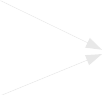Adding and subtracting fractions calculator
Calculator shows how to add or subtract two fractions step by step. Simply enter two fractions, select add or subtract and get list of all partial steps needed to compute result. Train your math with calculla!

# Diagram showing how to add (subtract) two fractions#

IIIIIIIVV
=
 1 1 4
=
 5 4
=
 5 ·3 4 ·3
=
 15 12=
 31 12
=
 2 7 12
=
 2 7 12
=
 1 1 3
=
 4 3
=
 4 ·4 3 ·4
=
 16 12

# Summary - add (subract) step by step#

StepsIIIIIIIVV
Summary
 1 1 4
+
 1 1 3
=
 5 4
+
 4 3
=
 5 ·3 4 ·3
=
 15 12
+
 16 12
=
 31 12
=
 2 7 12
=
 2 7 12

# How to use this tool#

Calculator shows how to add (subtract) two fractions. Enter input fractions and select needed operation (addition or subtraction) in form below and calculla will compute the results by performing following steps:
• I. Convert input fractions into improper form. If your fractions do not containt wholes part, then this step is unnecessary and can be skipped.
• II. Find common denominator. If input fractions already have common denominator, this step can be skipped.
• III. Perform operation. In this step, calculla adds (subtract) nominators and saves denominator without changes.
• IV. Get wholes part out of result. This step is needed only if result is improper fraction i.e. its nominator is greater than denominator.
• V. Shortening result to the simplest form.

# Tags and links to this website#

Tags:
Tags to Polish version:

# Permalink#

This is permalink. Permalink is the link containing your input data. Just copy it and share your work with friends:

# Ancient version of this site - links#

In December 2016 the Calculla website has been republished using new technologies and all calculators have been rewritten. Old version of the Calculla is still available through this link: v1.calculla.com. We left the version 1 of Calculla untouched for archival purposes.
Direct link to the old version:
"Calculla v1" version of this calculator
JavaScript failed !
So this is static version of this website.
This website works a lot better in JavaScript enabled browser.
Please enable JavaScript.